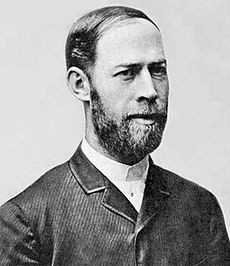# Why do technical terms sound so funny?Ohm. Hertz. Coulomb. Watt. These are measurements of electricity in its many forms. But they’re weird sounding, right? It’s not just me, right?

## Here’s why they’re called that

It’s been common for the last 150 years or so to name scientific measurements after someone prominent in the field. The names are actually the names of the scientists who first sought to understand the phenomena that’s being measured. A lot of them are tied together. Here’s just a few:

### Coulomb

Charles-Augustin de Coulomb discovered the relationship between the electrostatic forces of friction and repulsion, according to the internet. A coulomb is the amount of current conveyed by one ampere per second.

### Ampere

André-Marie Ampère created the entire field of electrodynamics.  An ampere, or amp, is a unit of current tied to a coulomb, useful in determining the overall draw of a device.

### Volt

Alessandra Volta invented the battery. A volt is a measure of elecromotive force and is helpful in determining how much power it takes to do something electrically.

### Watt

James Watt invented the modern steam engine. A watt is one joule per second, or can be determined by multiplying volts by amps.

### Joule

James Prescott Joule discovered the principle of conservation of energy, that energy is neither created nor destroyed. A joule is the amount of energy transferred when a force of one newton moves an object one meter (without resistance.)

### Newton

Isaac Newton was one of the most influential scientists in history and discovered the basic laws of motion. A newton is the force needed to accelerate one kilogram of mass at the rate of one meter per second squared.

### Hertz

Heinrich Hertz did some of the first pioneering work on broadcasting. A hertz equals one cycle per second.

### Bel

Alexander Graham Bell pioneered the transmission of voice and data over wires. It’s not clear if he actually invented the telephone, but either way his contributions to science are quite impressive. A bel is a measure of difference. 1 bel equals a tenfold increase. Most commonly a decibel (1/10th bel) is used because it is a more useful measure.

## As you see…

…for every measurement there is a story, and a pioneer for whom that measurement is named. It’s pretty neat if you think about it.